4WX Internet Search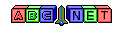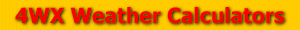# Density Altitude

From the user, an air temperature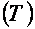, a station pressure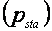, and a dewpoint temperature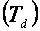The density altitude calculation is quite complex. Before calculating the density altitude, the virtual temperature must be calculated first. To perform all the calculations for the density altitude calculation, the air temperature must be in units of Kelvin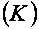, the station pressure must be in units of inches of mercury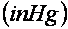and millibars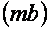, and the dewpoint temperature must be in units of degrees Celsius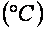To see how to convert the temperatures and pressure, see the links below:

tempConvert.php

To calculate the virtual temperature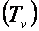, a vapor pressure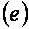must be calculated first using the equation below.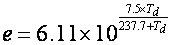Then using station pressure in units of millibars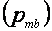, the virtual temperature can be calculated.The virtual temperature answer will be in units of Kevinand needs to be converted to Rankine to calculate the density altitude. To see how to convert Kelvin to Rankine, see the link above. Finally, the density altitude can be calculated. Using the virtual temperature in units of Rankineand station pressure in inches of mercury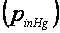The density altitude will be units of feet. To convert, the answer to meters, see the equation below: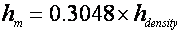Back to 4WX Weather Calculators index page

4WX Calculators Credit and disclaimer